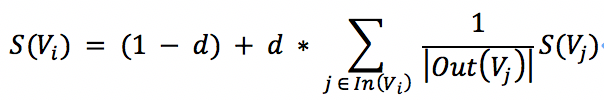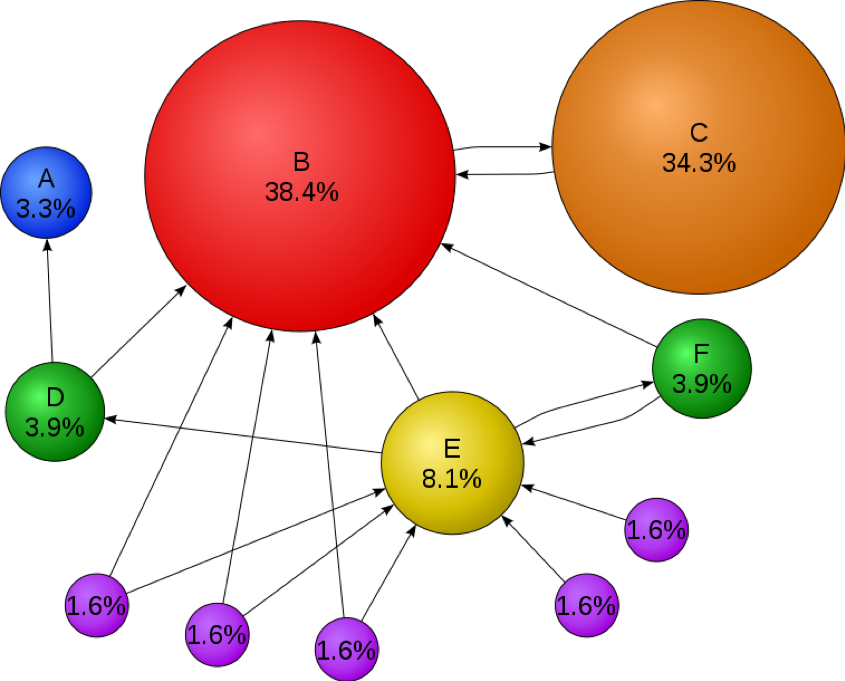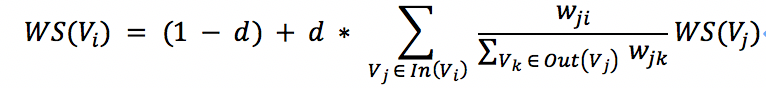# 简易中文自动文摘系统（四）：TextRank算法实现

## PageRank算法

PageRank算法输出一个概率分布，用于表示随机点击链接的人可能会到达任何特定页面的可能性。 可以为任意大小的文档集合计算PageRank。在一些研究论文中假定在计算过程开始时，分布在集合中的所有文档中均匀分布。PageRank计算需要多次通过，称为“迭代”，通过集合来调整近似的PageRank值，以更贴近地反映理论的真实值。

概率表示为介于0和1之间的数值。通常将0.5概率表示为发生某事的“50％几率”。 因此，0.5的PageRank意味着点击随机链接的人有50％的机会将被导向0.5 PageRank的文档。

那么根据以上设计思想，佩奇设计了下面的计算公式：这就是著名的PageRank算法公式。其中，S(V_i)是网页i的重要性(PR值)；d是阻尼系数，一般为0.85；In(V_i)是整个互联网中所存在的有指向网页i的链接的网页集合；Out(V_j)是网页j中存在的指向所有外部网页的链接的集合；|Out(V_j)|是该集合中元素的个数。output\input A B C D E F A 0 0 0 0 0 0 B 0 0 1 0 0 0 C 0 1 0 0 0 0 D 1 1 0 0 0 0 E 0 1 0 1 0 1 F 0 1 0 0 1 0

我们把这张表格称为交叉矩阵表，它反映了在一个互联网中，各个网页之间的链接关系，通过这个表格可以直接计算每张网页被其它网页引用的次数，在使用科学的公式进行计算，可以粗略算出这张网页在互联网上的重要程度。

## TextRank

TextRank是一种用于自然语言处理的通用基于图的排序算法。本质上，它在专门为特定NLP任务设计的图表上运行PageRank。对于关键词提取，它使用一些文本单元集作为顶点来构建图。边是基于文本单元顶点之间的语义或词汇相似度的度量。与PageRank不同的是，边缘通常是无向的，可以加权以反映相似程度。一旦图形被构建，它就被用来形成一个随机矩阵，结合一个阻尼因子(如在“随机冲浪模型”中)，并且通过找到对应于特征值1的特征向量来获得顶点的排名(即在图上随机游走的平稳分布)。

TextRank算法公式如下：其中，WS(V_i)表示句子i的权重(weight sum)，右侧的求和表示每个相邻句子对本句子的贡献程度。在单文档中，我们可以粗略认为所有句子都是相邻的，不需要像多文档一样进行多个窗口的生成和抽取，仅需单一文档窗口即可。求和的分母w_ji表示两个句子的相似度，而分母仍然表示权重，WS(V_j)代表上次迭代出的句子j的权重，因此，TextRank算法也是类似PageRank算法的、不断迭代的过程。

1.文本预处理

def cut_sentences(sentence):
puns = frozenset(u'.')
tmp = []
for ch in sentence:
tmp.append(ch)
if puns.__contains__(ch):
yield ''.join(tmp)
tmp = []
yield ''.join(tmp)

2.句子相似度计算

def two_sentences_similarity(sents_1, sents_2):
counter = 0
for sent in sents_1:
if sent in sents_2:
counter += 1
return counter / (math.log(len(sents_1) + len(sents_2)))

def cosine_similarity(vec1, vec2):
tx = np.array(vec1)
ty = np.array(vec2)
cos1 = np.sum(tx * ty)
cos21 = np.sqrt(sum(tx ** 2))
cos22 = np.sqrt(sum(ty ** 2))
cosine_value = cos1 / float(cos21 * cos22)
return cosine_value

def compute_similarity_by_avg(sents_1, sents_2):
if len(sents_1) == 0 or len(sents_2) == 0:
return 0.0
vec1 = model[sents_1]
for word1 in sents_1[1:]:
vec1 = vec1 + model[word1]
vec2 = model[sents_2]
for word2 in sents_2[1:]:
vec2 = vec2 + model[word2]
similarity = cosine_similarity(vec1 / len(sents_1), vec2 / len(sents_2))
return similarity

3.句子权重计算

def calculate_score(weight_graph, scores, i):
length = len(weight_graph)
d = 0.85
for j in range(length):
denominator = 0.0
fraction = weight_graph[j][i] * scores[j]
for k in range(length):
denominator += weight_graph[j][k]
if denominator == 0:
denominator = 1
weighted_score = (1 - d) + d * added_score
return weighted_score

4.句子排序

def weight_sentences_rank(weight_graph):
scores = [0.5 for _ in range(len(weight_graph))]
old_scores = [0.0 for _ in range(len(weight_graph))]
while different(scores, old_scores):
for i in range(len(weight_graph)):
old_scores[i] = scores[i]
for i in range(len(weight_graph)):
scores[i] = calculate_score(weight_graph, scores, i)
return scores

5.生成文摘

def summarize(text, n):
tokens = cut_sentences(text)
sentences = []
sents = []
for sent in tokens:
sentences.append(sent)
sents.append([word for word in jieba.cut(sent) if word])
sents = filter_model(sents)
graph = create_graph(sents)
scores = weight_sentences_rank(graph)
sent_selected = nlargest(n, zip(scores, count()))
sent_index = []
for i in range(n):
sent_index.append(sent_selected[i])
return [sentences[i] for i in sent_index]

if __name__ == '__main__':
with open("njuptcs.txt", "r") as njuptcs:
txt = [line.strip() for line in open("njuptcs.txt", "r").readlines()]
print '原文为：'
for i in txt:
print i
summarize_text = summarize(text, 5)
print '摘要为：'
for i in summarize_text:
print i12-232045
07-102103
02-141万+
07-103339
07-142万+
01-036184
11-159191
08-311万+
07-302036
01-21562
09-07679
05-182万+
07-301489
07-103208
07-102302
05-234020